# Top 10 Machine Learning Algorithms For Beginners

Alan Turing, an English mathematician, computer scientist, logician, and cryptanalyst, surmised about machines that,

“It would be like a pupil who had learnt much from his master but had added much more by his own work. When this happens I feel that one is obliged to regard the machine as showing intelligence."

To give you an example of the impact of machine learning, Man group’s AHL Dimension programme is a \$5.1 billion dollar hedge fund which is partially managed by AI. After it started off, by the year 2015, its machine learning algorithms were contributing more than half of the profits of the fund even though the assets under its management were far less.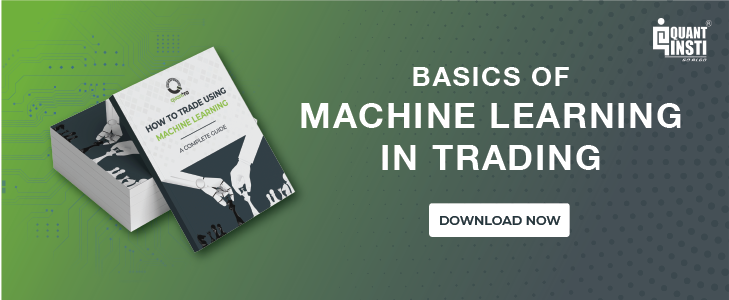After reading this blog, you would be able to understand the basic logic behind some popular and incredibly resourceful machine learning algorithms which have been used by the trading community as well as serve as the foundation stone on which you step on to create the best machine learning algorithm. They are:

## Linear Regression

Initially developed in statistics to study the relationship between input and output numerical variables, it was adopted by the machine learning community to make predictions based on the linear regression equation.

The mathematical representation of linear regression is a linear equation that combines a specific set of input data (x) to predict the output value (y) for that set of input values. The linear equation assigns a factor to each set of input values, which are called the coefficients represented by the Greek letter Beta (β).

The equation mentioned below represents a linear regression model with two sets of input values, x1 and x2. y represents the output of the model, β0, β1 and β2 are the coefficients of the linear equation.

y = β0 + β1x1 + β2x2

When there is only one input variable, the linear equation represents a straight line. For simplicity, consider β2 to be equal to zero, which would imply that the variable x2 will not influence the output of the linear regression model. In this case, the linear regression will represent a straight line and its equation is shown below.

y = β0 + β1x1

A graph of the linear regression equation model is as shown below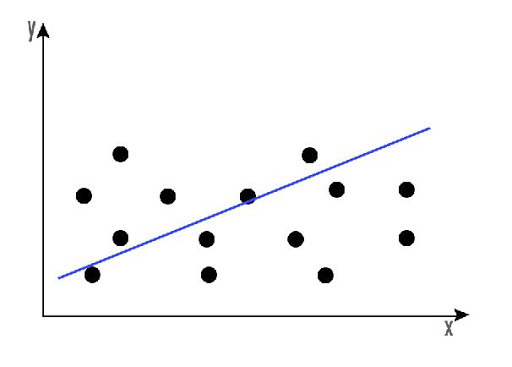Linear regression can be used to find the general price trend of a stock over a period of time. This helps us understand if the price movement is positive or negative.

## Logistic regression

In logistic regression, our aim is to produce a discrete value, either 1 or 0. This helps us in finding a definite answer to our scenario.

Logistic regression can be mathematically represented as,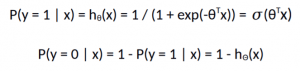The logistic regression model computes a weighted sum of the input variables similar to the linear regression, but it runs the result through a special non-linear function, the logistic function or sigmoid function to produce the output y.

The sigmoid/logistic function is given by the following equation.

y = 1 / (1+ e-x)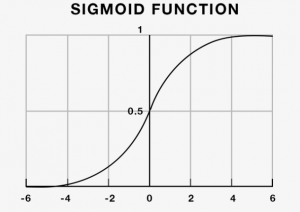In simple terms, logistic regression can be used to predict the direction of the market.

## KNN Classification

The purpose of the K nearest neighbours (KNN) classification is to separate the data points into different classes so that we can classify them based on similarity measures (e.g. distance function).

KNN learns as it goes, in the sense, it does not need an explicit training phase and starts classifying the data points decided by a majority vote of its neighbours.

The object is assigned to the class which is most common among its k nearest neighbours.

Let’s consider the task of classifying a green circle into class 1 and class 2. Consider the case of KNN based on 1-nearest neighbour. In this case, KNN will classify the green circle into class 1. Now let’s increase the number of nearest neighbours to 3 i.e., 3-nearest neighbour. As you can see in the figure there are ‘two’ class 2 objects and ‘one’ class 1 object inside the circle. KNN will classify a green circle into class 2 object as it forms the majority.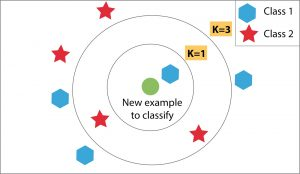## Support Vector Machine (SVM)

Support Vector Machine was initially used for data analysis. Initially, a set of training examples is fed into the SVM algorithm, belonging to one or the other category. The algorithm then builds a model that starts assigning new data to one of the categories that it has learned in the training phase.

In the SVM algorithm, a hyperplane is created which serves as a demarcation between the categories. When the SVM algorithm processes a new data point and depending on the side on which it appears it will be classified into one of the classes.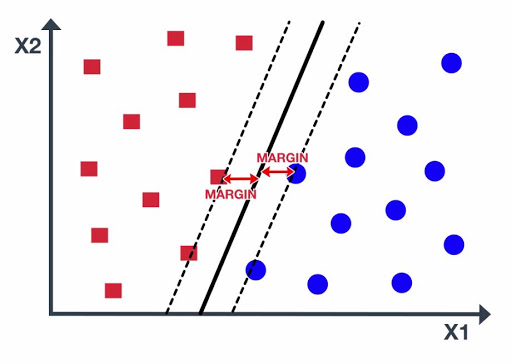When related to trading, an SVM algorithm can be built which categorises the equity data as a favourable buy, sell or neutral classes and then classifies the test data according to the rules.

## Decision Trees

Decision trees are basically a tree-like support tool which can be used to represent a cause and its effect. Since one cause can have multiple effects, we list them down (quite like a tree with its branches).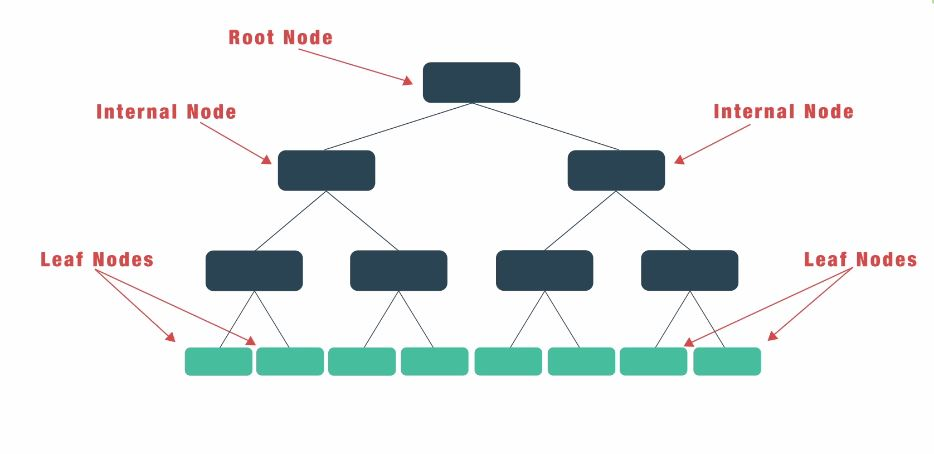We can build the decision tree by organising the input data and predictor variables, and according to some criteria that we will specify.

The main steps to build a decision tree are:

1. Retrieve market data for a financial instrument.
2. Introduce the Predictor variables (i.e. Technical indicators, Sentiment indicators, Breadth indicators, etc.)
3. Setup the Target variable or the desired output.
4. Split data between training and test data.
5. Generate the decision tree training the model.
6. Testing and analyzing the model.

The disadvantage of decision trees is that they are prone to overfitting due to their inherent design structure.

## Random Forest

A random forest algorithm was designed to address some of the limitations of decision trees.

Random Forest comprises of decision trees which are graphs of decisions representing their course of action or statistical probability. These multiple trees are mapped to a single tree which is called Classification and Regression (CART) Model.

To classify an object based on its attributes, each tree gives a classification which is said to “vote” for that class. The forest then chooses the classification with the greatest number of votes. For regression, it considers the average of the outputs of different trees.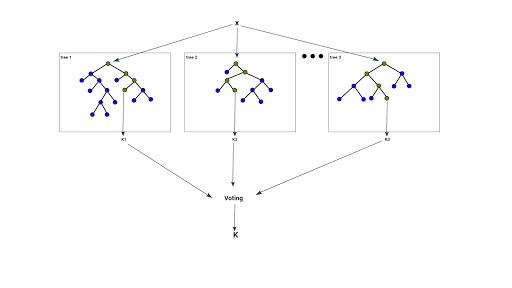Random Forest works in the following way:

1. Assume the number of cases as N. A sample of these N cases is taken as the training set.
2. Consider M to be the number of input variables, a number m is selected such that m < M. The best split between m and M is used to split the node. The value of m is held constant as the trees are grown.
3. Each tree is grown as large as possible.
4. By aggregating the predictions of n trees (i.e., majority votes for classification, average for regression), predict the new data.

## Artificial Neural Network

In our quest to play God, an artificial neural network is one of our crowning achievements. We have created multiple nodes which are interconnected to each other, as shown in the image, which mimics the neurons in our brain. In simple terms, each neuron takes in information through another neuron, performs work on it, and transfers it to another neuron as output.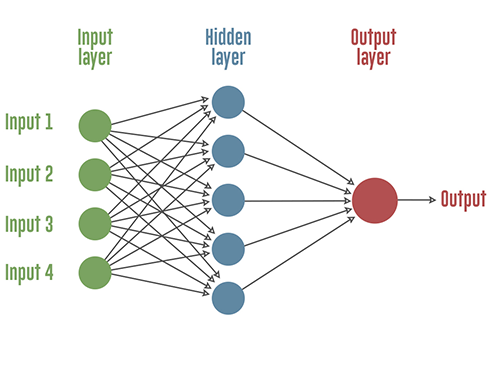Each circular node represents an artificial neuron and an arrow represents a connection from the output of one neuron to the input of another.

Neural networks can be more useful if we use it to find interdependencies between various asset classes, rather than trying to predict a buy or sell choice.

## K-means Clustering

In this machine learning algorithm, the goal is to label the data points according to their similarity. Thus, we do not define the clusters prior to the algorithm but instead, the algorithm finds these clusters as it goes forward.

A simple example would be that given the data of football players, we will use K-means clustering and label them according to their similarity. Thus, these clusters could be based on the strikers preference to score on free kicks or successful tackles, even when the algorithm is not given pre-defined labels to start with.

K-means clustering would be beneficial to traders who feel that there might be similarities between different assets which cannot be seen on the surface.

## Naive Bayes theorem

Now, if you remember basic probability, you would know that Bayes theorem was formulated in a way where we assume we have prior knowledge of any event that related to the former event.

For example, to check the probability that you will be late to the office, one would like to know if you face any traffic on the way.

However, Naive Bayes classifier algorithm assumes that two events are independent of each other and thus, this simplifies the calculations to a large extent. Initially thought of nothing more than an academic exercise, Naive Bayes has shown that it works remarkably well in the real world as well.

Naive Bayes algorithm can be used to find simple relationships between different parameters without having complete data.

## Recurrent Neural Networks (RNN)

Did you know Siri and Google Assistant use RNN in their programming? RNNs are essentially a type of neural network which have a memory attached to each node which makes it easy to process sequential data i.e. one data unit is dependent on the previous one.

A way to explain the advantage of RNN over a normal neural network is that we are supposed to process a word character by character. If the word is “trading”, a normal neural network node would forget the character “t” by the time it moves to “d” whereas a recurrent neural network will remember the character as it has its own memory.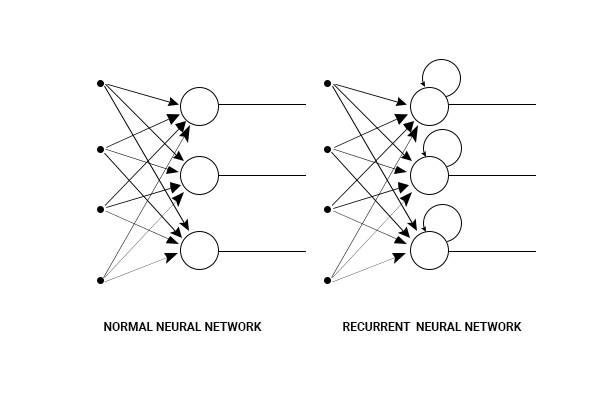## Conclusion

According to a study by Preqin, 1,360 quantitative funds are known to use computer models in their trading process, representing 9% of all funds. Firms organise cash prizes for an individual's machine learning strategy if it makes money in the test phase and in fact, invest their own money and take it in the live trading phase. Thus, in the race to be one step ahead of the competition, everyone, be it billion dollar hedge funds or the individual trade, all are trying to understand and implement machine learning in their trading strategies.

You can go through the AI in Trading course on Quantra to learn these algorithms in detail as well as apply them in live markets successfully and efficiently.

You can enroll for the online machine learning course on Quantra which covers classification algorithms, performance measures in machine learning, hyper-parameters, and building of supervised classifiers.